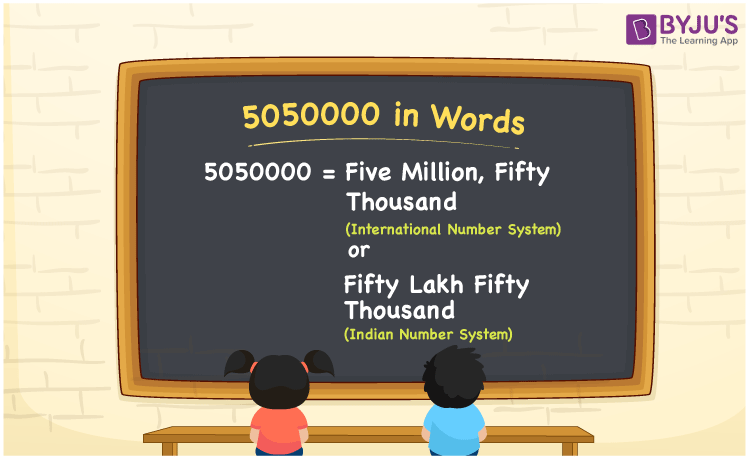# 5050000 in Words

The number name of 5050000 as per the Indian Number System is “Fifty lakh fifty thousand”. Whereas in the International Number System, the number 5050000 in words is “Five million and fifty thousand”. Now, let us learn the procedure of writing the number 5050000 in words using the place value system in detail.

 5050000 in Words (Indian Number System): Fifty lakh fifty thousand  5050000 in Words (International Number System): Five Million and Fifty Thousand Fifty Lakh Fifty Thousand in Numerical Form: 5050000.

## 5050000 in English Words## How to Write 5050000 in Words?

Following is the place value table for the number 5050000:

 Ten-lakhs Lakhs Ten-thousands Thousands Hundreds Tens Ones 5 0 5 0 0 0 0

The expanded form of 5050000 is as follows:

= 5 × Ten lakh + 0 × lakh + 5 × Ten thousand + 0 × Thousand + 0 × Hundred + 0 × Ten + 0 × One

= 5 × 1000000 + 0 × 100000 + 5 × 10000 + 0 × 1000 + 0 × 100 + 0 × 10 + 0 × 1

= 5000000 + 50000

= 5050000

= Fifty lakh fifty thousand

Hence, 5050000 in words is fifty lakh fifty thousand (or) five million and fifty thousand.

5050000 in words – Fifty lakh fifty thousand (or) five million and fifty thousand

Is 5050000 an odd number? – No

Is 5050000 an even number? – Yes

Is 5050000 a perfect square number? – No

Is 5050000 a perfect cube number? – No

Is 5050000 a prime number? – No

Is 5050000 a composite number? – Yes

## Frequently Asked Questions on 5050000 in Words

Q1

### How to write 5050000 in words as per the Indian Number System?

According to the Indian Number System, 5050000 in words is fifty lakh fifty thousand.

Q2

### Simplify 5000000 + 50000, and express it in words.

Simplifying 5000000 + 50000, we get 5050000. Hence, 5050000 in words is fifty lakh fifty thousand.

Q3

### How to write 5050000 in words as per the International Number System?

According to the International Number System, 5050000 in words is five million and fifty thousand